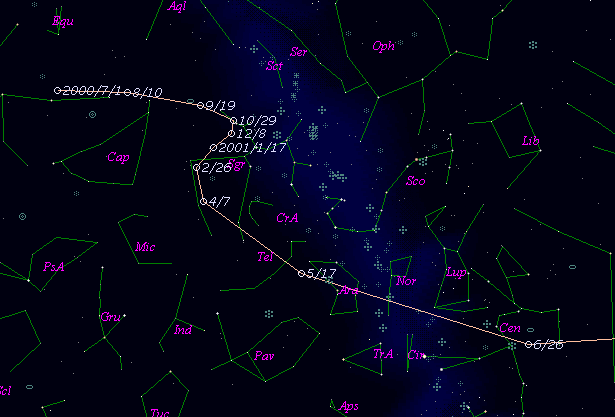# \$B%9%Z!<%9%&%)%C%AWB@1(B

C/2000 OF8 ( Spacewatch )###\$B%W%m%U%#!<%k(B

 \$BH/8+F|(B 2000\$BG/(B7\$B7n(B24\$BF|(B \$BH/8+8wEY(B 21.3\$BEy(B \$BH/8+ A. Descour, J. Larsen, R. S. McMillan, J. V. Scotti (Steward Observatory, Kitt Peak)

###\$B50F;MWAG(B

```   The following improved orbital elements by Kenji Muraoka, are
from 55 observations 2000 July 24 to 2001 Apr. 23, perturbations
by 9 Planets, Moon and 5 minor planets were taken into account.
The mean residual is +/- 0.48 arc seconds.

Epoch  =  2001 July 30.0  TT       JDT = 2452120.5
T  =  2001 Aug.  4.77375       +/- 0.00187 (m.e.) TT
Peri. =  256.06697                +/- 0.00148
Node  =  117.09207                +/- 0.00172   (2000.0)
Incl. =  152.43656                +/- 0.00114
q  =    2.1731102              +/- 0.0000725 AU
e  =    1.0018143              +/- 0.0000124
1/a  =   -0.0008349              +/- 0.0000057 1/AU
orig. 1/a  =   +0.0000469
fut.  1/a  =   -0.0025352
```

###\$B@1?^(B###\$B8wEYJQ2=(B

```        m1 = 10.0 + 5 log\$B&\$(B + 10.0 log r
```##### \$B50F;MWAG\$OB<2,7r<#;a\$N7W;;\$K\$h\$k\$b\$N\$G\$9!#(B \$B@1?^\$O(B StellaNavigator Ver.2.0 for Windows (\$B%"%9%H%m%"!<%D(B \$BJTCx(B / \$B%"%9%-!<=PHG6I4)(B) \$B\$G:n@.\$7\$?\$b\$N\$G\$9!#(B \$B8wEY%0%i%U\$O(BComet for Windows\$B\$G:n@.\$7\$?\$b\$N\$G\$9!#(B